# 1、引言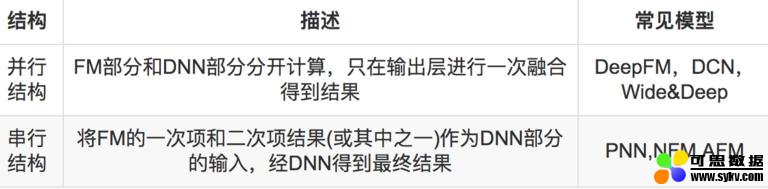# 2、AFM 模型介绍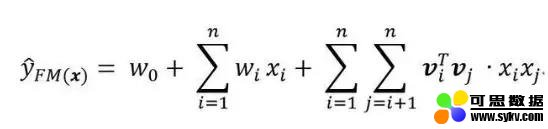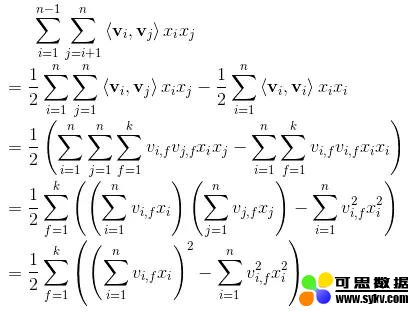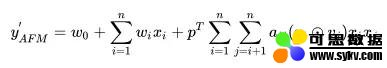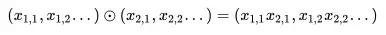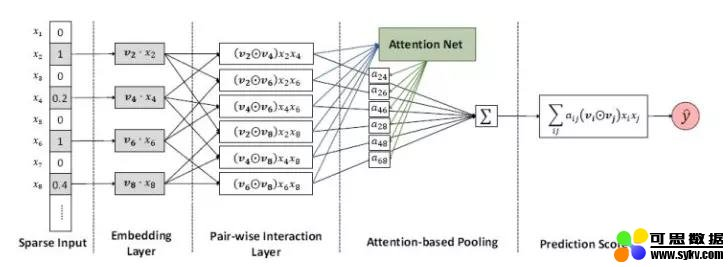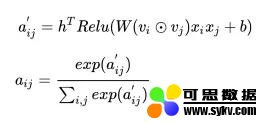# 3、代码实现

https://github.com/princewen/tensorflow_practice/tree/master/recommendation/Basic-AFM-Demo

Embedding Size：K

Batch Size：N

Attention Size ：A

Field Size ( 这里是 field size 不是 feature size！！！！）： F

• 本文地址：http://www.6aiq.com/article/1547806920160
• 本文版权归作者和AIQ共有，欢迎转载，但未经作者同意必须保留此段声明，且在文章页面明显位置给出
• 知乎专栏 点击关注

``````self.feat_index = tf.placeholder(tf.int32,
shape=[None,None],
name='feat_index')
self.feat_value = tf.placeholder(tf.float32,
shape=[None,None],
name='feat_value')

self.label = tf.placeholder(tf.float32,shape=[None,1],name='label')
self.dropout_keep_deep = tf.placeholder(tf.float32,shape=[None],name='dropout_deep_deep')

``````

feat_index是特征的一个序号，主要用于通过 embedding_lookup 选择我们的 embedding。feat_value是对应的特征值，如果是离散特征的话，就是 1，如果不是离散特征的话，就保留原来的特征值。label 是实际值。还定义了 dropout 来防止过拟合。

``````def _initialize_weights(self):
weights = dict()

#embeddings
weights['feature_embeddings'] = tf.Variable(
tf.random_normal([self.feature_size,self.embedding_size],0.0,0.01),
name='feature_embeddings')
weights['feature_bias'] = tf.Variable(tf.random_normal([self.feature_size,1],0.0,1.0),name='feature_bias')
weights['bias'] = tf.Variable(tf.constant(0.1),name='bias')

``````

Attention 部分的权重我们详细介绍一下，这里共有四个部分，分别对应公式中的 w，b，h 和 p。

weights[‘attention_w’] 的维度为 K * A，

weights[‘attention_b’] 的维度为 A，

weights[‘attention_h’] 的维度为 A，

weights[‘attention_p’] 的维度为 K * 1

``````# attention part
glorot = np.sqrt(2.0 / (self.attention_size + self.embedding_size))

weights['attention_w'] = tf.Variable(np.random.normal(loc=0,scale=glorot,size=(self.embedding_size,self.attention_size)),
dtype=tf.float32,name='attention_w')

weights['attention_b'] = tf.Variable(np.random.normal(loc=0,scale=glorot,size=(self.attention_size,)),
dtype=tf.float32,name='attention_b')

weights['attention_h'] = tf.Variable(np.random.normal(loc=0,scale=1,size=(self.attention_size,)),
dtype=tf.float32,name='attention_h')

weights['attention_p'] = tf.Variable(np.ones((self.embedding_size,1)),dtype=np.float32)
``````

Embedding Layer

``````# Embeddings
self.embeddings = tf.nn.embedding_lookup(self.weights['feature_embeddings'],self.feat_index) # N * F * K
feat_value = tf.reshape(self.feat_value,shape=[-1,self.field_size,1])
self.embeddings = tf.multiply(self.embeddings,feat_value) # N * F * K

``````

Attention Net
Attention 部分的实现严格按照上面给出的数学公式：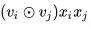``````element_wise_product_list = []
for i in range(self.field_size):
for j in range(i+1,self.field_size):
element_wise_product_list.append(tf.multiply(self.embeddings[:,i,:],self.embeddings[:,j,:])) # None * K

self.element_wise_product = tf.stack(element_wise_product_list) # (F * F - 1 / 2) * None * K
self.element_wise_product = tf.transpose(self.element_wise_product,perm=[1,0,2],name='element_wise_product') # None * (F * F - 1 / 2) *  K

``````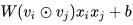``````self.attention_wx_plus_b = tf.reshape(tf.add(tf.matmul(tf.reshape(self.element_wise_product,shape=(-1,self.embedding_size)),
self.weights['attention_w']),
self.weights['attention_b']),
shape=[-1,num_interactions,self.attention_size]) # N * ( F * F - 1 / 2) * A

``````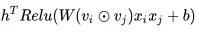``````self.attention_exp = tf.exp(tf.reduce_sum(tf.multiply(tf.nn.relu(self.attention_wx_plus_b),
self.weights['attention_h']),
axis=2,keep_dims=True)) # N * ( F * F - 1 / 2) * 1
``````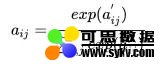``````self.attention_exp_sum = tf.reduce_sum(self.attention_exp,axis=1,keep_dims=True) # N * 1 * 1

self.attention_out = tf.div(self.attention_exp,self.attention_exp_sum,name='attention_out') # N * ( F * F - 1 / 2) * 1

``````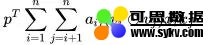``````self.attention_x_product = tf.reduce_sum(tf.multiply(self.attention_out,self.element_wise_product),axis=1,name='afm') # N * K

self.attention_part_sum = tf.matmul(self.attention_x_product,self.weights['attention_p']) # N * 1

``````

``````# first order term
self.y_first_order = tf.nn.embedding_lookup(self.weights['feature_bias'], self.feat_index)
self.y_first_order = tf.reduce_sum(tf.multiply(self.y_first_order, feat_value), 2)

# bias
self.y_bias = self.weights['bias'] * tf.ones_like(self.label)
``````

``````# out
self.attention_part_sum,
self.y_bias],name='out_afm')

``````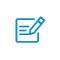扫码入群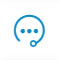咨询反馈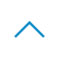返回顶部